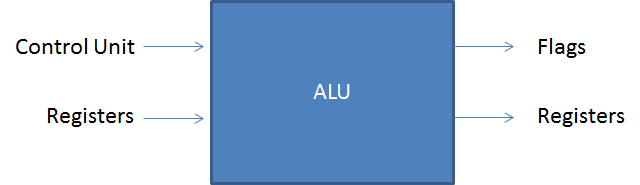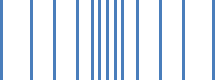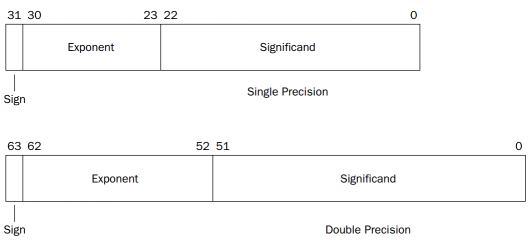Geeks With Blogs

Learning Outcomes

• Explain how integers can be represented in a computer
• Use assembly language to perform arithmetic and logical operations on integers on a Pentium
• Use assembly language to perform bit manipulation operations on a Pentium
• Explain how floating point numbers are stored in a computer
• Show how a floating point number is stored in a computer using a given gloating point format
• calculate the value of a floating point number that is stored in a computer given the relevant floating point format

The Arithmetic and Logic Unit (ALU)

• The ALU performs arithmetic and logical operations on data
• The ALU is in essence the core of a computerIn the diagram above the general terms of an ALU are represented and connected with the rest of the processor…

• Data is presented to the ALU in registers
• The results of the operation are stored in registers (these registers are temporary storage locations within the processor that are connected by signal paths to the ALU)
• The ALU may set flags as a result of an operations (i.e. overflow)
• The control unit provides signals that control the operation of the ALU and the movement of the data into and out of the ALU

Integer Representation

There are a number of different ways in which integers can be represented on a computer including sign-magnitude and two complement.

Sign Magnitude Representation

With sign magnitude representation the leftmost significant bit in the word represents a sign

• 0 represents positive numbers

For example…

+18 = 00010010 while -18 = 10010010 (the leftmost bit represents the sign)

There are several drawbacks to sign-magnitude representation including…

• Addition and subtraction require a consideration of both the signs of the numbers and their relative magnitudes to carry out the required operation
• There are two representations of 0 (00000000 and 10000000) which makes it slightly harder to test for zero

Sign magnitude representation is rarely used in implementing the integer portion of the ALU. The alternative and most common schema is two’s complement representation.

Two’s Complement Representation

• Two’s complement representation uses the most significant bit as a sign bit.
• It differs from sign-magnitude in the way that the other bits are interpreted
• Most treatments of twos complement representation focus on the rules for producing negative numbers

To convert a number from sign magnitude to two’s complement you can perform the following steps…

If it is a positive number…

• it is represented as a normal binary number

If it is a negative number…

• take the number in sign magnitude and inverse the 1’s and 0’s
• add 1 to the total
• the number is represented in two’s complement

e.g take the number 7 & –7 represented in 4 bits…

In sign magnitude it is represented as follows…

• 7 = 0111
• -7 = 1111

Two’s complement of the same numbers are as follows…

• 7 = 0111 (no conversion necessary)
• -7 = 0111 (inversed) = 1000 (add 0001 to it) = 1001

Converting between different bit lengths

One challenge with two’s complement is converting negative numbers to increase the bit lengths. You cannot just move the sign. Instead apply the following process…

• move the sign bit to the new leftmost position
• fill in the spaces with copies of the sign bit (for positive numbers fill in with 0’s and for negative numbers fill in with 1’s)

.e.g. –18 = 11101110 (8 bits) & –18 = 1111111111101110 (16 bits)

Integer Arithmetic

I am only going to summarize two’s complement for these functions – the sign magnitude is relatively simple…

Negation

Negating a number can be done as follows…

• Take the Boolean complement of each bit of the integer (including the sign bit)
• Treating the result as an unsigned binary integer, add 1

e.g. 18 = 00010010 (2’s complement) = 11101101 (inverse or bitwise complement) + 1 = 11101110 (-18 twos complement) = –18

To make –18 equal 18 do the following…

e.g. –18 = 11101110 = 00010001 + 1 = 00010010 = 18

Two fringe cases you need to be aware of…

• what happens when we negate 0? = 00000000 = 11111111 + 1 = 0000000 (carry 1)
• what happens when we negate 10000000? = 01111111 + 1 = 10000000 (which means we are back to the original number which is not desired, this is because we are on the bounds of the range)

• Addition proceeds as if the two numbers were unsigned integers
• If both numbers are positive, we will end up with a positive result
• If the result of the operation is negative, we get a negative number in twos complement form

e.g. (1) 0001 + (1) 0001 = (2) 0010 (positive + positive)

keep in mind that -1 in 2’s complement is represented as follows 0001 + (bitwise complement)  + 1 = 1110 + 1 = 1111

so….

e.g. (1) 0001 + (-2) 1110  =  (-1) 1111 (-1)

On any addition, the result may be larger than can be held in the word size being used. This condition is called overflow. When overflow occurs, the ALU must signal this fact so that no attempt is made to use the result.

The overflow rule is as follows…

• If two numbers are added, and they are both positive or both negative, then overflow occurs if and only if the result has the opposite sign

Subtraction

Subtraction is performed exactly the same way as addition. In fact the example of 1 + (-2) could also be represented as 1 – 2.

Floating Point Representation

With fixed point notation it is possible to represent a range of positive and negative integers centred on 0. By assuming a fixed binary or radix point, this format allows the representation of numbers with a fractional component as well however the approach has limitations – very large numbers and very small fractions cannot be represented. To allow for such numbers we used floating point numbers.

A floating point number has 3 fields

• Sign: plus or minus
• Significand
• Exponent

It would look something like this…

 Sign (1 bit) 8 bits 23 bits s e (Biased exponent) f (Significand)

To get the value of a number given a 32 bit bit pattern we use the following formula:

value = (-1)^s 1.f x 2^(e-127)

Keep in mind the number is normalized – meaning the number is one in which the most significant digit of the significand is nonzero (for binary this means it must be 1).

When actually representing this number, the bit normalized bit is not necessary to show as it would always be one, and so it is omitted.

See the following example…

represent –7.6875 in IEEE floating point format…

7.6875 (base 10)

= 111.1011 (base 2)

= 1.111011 (base 2) * 2^2 (normalize)

= 1.111011 (base 2) * 2^(129-127)

= 1.111011 (base 2) * 2^(10000001 – 127)

 sign 8 bits 23 bits 1 10000001 1110110…..0

Note – with floating point numbers we are not able to represent more numbers than with fixed point numbers – we can basically represent about the same amount of numbers – the difference is their spread. With fixed point numbers, each number represented is evenly spread across the number line. With floating point numbers as you get closer to zero, the numbers get closer together, as you get larger or smaller than zero the spread increases.The main standard for floating point numbers is the IEEE Standard. It looks as follows…There are other standards, but the IEEE standard is the dominant one. Others mentioned in the notes include…

• The IBM 306/370 format
• The DEC PDP 11/VAX Format
Posted on Tuesday, March 13, 2012 6:14 AM UNISA COS 2621 Computer Organization | Back to top

Related Posts on Geeks With Blogs Matching Categories

Comments on this post: Organization & Architecture UNISA Studies - Chap 9

No comments posted yet.